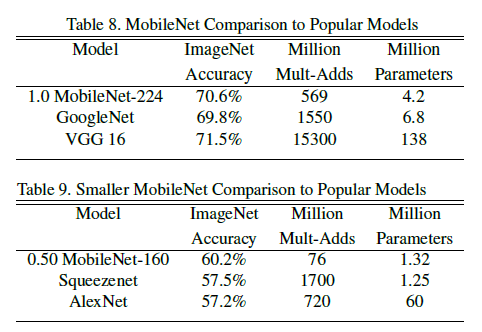# 1. 概述

MobileNets: Efficient Convolutional Neural Networks for Mobile Vision Applications 一文发表于 2017 年，文章提出了一种适合移动端和嵌入式平台的高效卷积网络，称为 MobbileNets，并引入了两个全局超参数，用于调整模型体积，在延迟和准确率之间进行取舍。

构建小型模型的方法主要有两种：一种方法是通过如量化、哈希、剪枝等方法，对预训练模型进行压缩；另一种方法是直接训练小模型。MobileNet 属于后者，它的主要特点是使用深度可分离卷积（depthwise separable
convolution）代替了普通卷积，大幅降低了计算量。MobileNet 的主要目标是优化延迟，同时减小了模型体积。

# 2. 标准卷积

标准卷积层一般使用一个 $D_K \times D_K \times M \times N$ 的卷积核 $K$ 对图像进处理，其中 $D_K$ 是过滤器的尺寸（通常是正方形），$M$ 是输入通道数量，$N$ 是输出通道数量，如 Figure 2(a)。

步长为 $1$ 且使用 padding 时，标准卷积输出的特征图为

\begin{equation}
G_{k, l, n} = \sum_{i, j, m} K_{i, j, m, n} \cdot F_{k+i-1, l+j-1, m} \tag{1}
\end{equation}

\begin{equation}
D_K \cdot D_K \cdot M \cdot N \cdot D_F \cdot D_F \tag{2}
\end{equation}

标准卷积一方面通过卷积核对特征进行过滤，同时将这些特征组合起来，产生新的表示。过滤和组合的操作只需要一层就可以完成。

# 3. 深度可分离卷积

深度可分离卷积是一种分解卷积（factorized convolution），它将一个普通的卷积分解为一个 depthwise 卷积，以及一个称为 pointwise 卷积的 $1 \times 1$ 卷积。普通卷积只需要一层就可以同时进行过滤和组合的操作，而深度可分离卷积将这些操作分在两层进行：一层进行过滤，一层进行组合。

具体来说，MobileNet 中的深度可分离卷积先通过一层 depthwise 卷积使用单个过滤器作用在每个输入通道上，然后再通过一层 $1 \times 1$ 的 pointwise 卷积计算 depthwise 卷积结果的一个线性组合。此外 MobileNet 的对这两类层都使用了 BatchNorm 和 ReLU 激活函数。

depthwise 卷积使用 $D_K \times D_K \times M$ 的卷积核，如 Figure 2(b)，计算可以写为

\begin{equation}
\hat{G}_{k, l, m} = \sum_{i, j} \hat{K}_{i, j, m} \cdot F_{k+i-1, l+j-m} \tag{3}
\end{equation}

\begin{equation}
D_K \cdot D_K \cdot M \cdot D_F \cdot D_F \tag{4}
\end{equation}

$1 \times 1$ pointwise 卷积使用 $1 \times 1 \times M \times N$ 的卷积核，如 Figure 2(c)，相当于计算了 depthwise 卷积结果的一个线性组合，不改变特征图尺寸，只改变通道数量。计算量为

\begin{equation}
M \cdot N \cdot D_F \cdot D_F
\end{equation}

depthwise 和 $1 \times 1$ pointwise 卷积结合起来，称为深度可分离卷积，总计算量为

\begin{equation}
D_K \cdot D_K \cdot M \cdot D_F \cdot D_F + M \cdot N \cdot D_F \cdot D_F \tag{5}
\end{equation}

\begin{equation}
\frac{D_K \cdot D_K \cdot M \cdot D_F \cdot D_F + M \cdot N \cdot D_F \cdot D_F}{D_K \cdot D_K \cdot M \cdot N \cdot D_F \cdot D_F} = \frac{1}{N} + \frac{1}{D_K^2}
\end{equation}

MobileNet 使用 $3 \times 3$ 的深度可分离卷积，计算量只有标准卷积的 $\frac{1}{8}$ 到 $\frac{1}{9}$，准确率只有微小降低。

# 4. MobileNet 网络结构

## 4.1. 网络结构

MobileNet 的网络结构如 Table 1。

## 4.2. 计算实现

文章指出，在构建高性能网络时，单纯追求缩减乘加数量是不够的，还要考虑计算操作的高效实现。由 Table 1 可见，深度可分离卷积输出的通道数（$N$）远大于 depthwise 卷积过滤器尺寸（$D_K$ \times $D_K$，即 $3 \times 3$），由式 $5$ 可知，此时大部分计算量发生在 $1 \times 1$ 卷积阶段。实际上，MobileNet 中 $1 \times 1$ 卷积占用了 95% 的计算和 $75$ 的参数，如 Table 2。

$1 \times 1$ 卷积可以通过高度优化的通用矩阵乘法（General Matrix Multiply，GEMM）函数完成。对于标准卷积，在使用 GEMM 计算前需要先通过 im2col 对数据在内存中进行重新排列，而 $1 \times 1$ 卷积则不需要重排这一步，可以直接通过 GEMM 实现，从而进一步提高了性能。

## 4.3. 训练

文章给出的 MobileNet 在 TensorFlow 上使用异步梯度下降的 RMSProp 训练。因为 MobileNet 很小，不容易出现过拟合，可以使用更少的正则化和数据增强。对于 depthwise 卷积，其参数尤其少，可以不使用或者使用非常小的权重衰减。

## 4.4. 宽度乘数

除了 MobileNet 本身，文章还给出了两个超参数，用来进一步调整网络尺寸。宽度乘数（Width Multiplier）$\alpha$ 可以统一调整各层的宽度（通道数），对于 $M$ 个输入通道和 $N 个输出通道$，调整后变为 $\alpha M$ 个输入通道和 $\alpha N$ 个输出通道，计算量变为

\begin{equation}
D_K \cdot D_K \cdot \alpha M \cdot D_F \cdot D_F + \alpha M \cdot \alpha N \cdot D_F \cdot D_F \tag{6}
\end{equation}

## 4.5. 分辨率乘数

分辨率乘数 $\rho$ 用于调整输入图像分辨率，以及网络每一层中的特征图尺寸。此时计算量变为

\begin{equation}
D_K \cdot D_K \cdot \alpha M \cdot \rho D_F \cdot \rho D_F + \alpha M \cdot \alpha N \cdot \rho D_F \cdot \rho D_F \tag{7}
\end{equation}

# 5. 实验结果

文章给出了各种缩放方式实验结果。Table 4 对比了使用标准卷积和深度可分离卷积的 MobileNet，可见深度可分离卷积相比标准卷积，大幅降低了计算量和参数数量，准确率只有 1% 的降低。

Table 5 对比了使用 0.75 宽度乘数的 MobileNet 和使用了更少层数的 MobileNet，可见在相近计算量和参数数量的情况下，通过宽度缩放网络的准确率要优于单纯降低网络层数。

Table 6 和 Table 7 分别对比了使用宽度和分辨率乘数缩放网络的效果，乘数越小，计算量和参数数量越少，准确率也随之下降，可以根据实际需要选择不同的缩放方式和等级。注意宽度乘数为 $0.25$ 时，模型变得过小，准确率出现了大幅下降。

文章还给出了 MobileNet 和其他模型的对比结果。Table 8 使用 MobileNet 和当时流行模型进行对比，Table 9 使用缩小的 MobileNet（$\alpha = 0.5$，分辨率为 $160 \times 160$ ）和其他模型进行对比。可见相比其他模型，MobileNet 在达到相近或更高准确率的同时，大幅降低了计算量和参数数量。

文章还给出了 MobileNet 在其他任务上的性能，包括细粒度的 Stanford Dogs 数据，地理定位任务（geolocalizaton），人脸属性（face attributes）、物体检测（object detection）以及人脸嵌入（face embeddings），详细数据可以参考原文。

值得一提的是，在人脸属性任务中，文章使用了蒸馏（distillation）的方法来训练模型，训练分类器模拟另一个更大的模型的输出，而不是直接使用带标签的数据进行训练。由于 MobileNet 参数很少，训练过程中不需要使用权重衰减或者提前停止等正则化方法，而且训练得到 MobileNet 在具有更少计算和参数的情况下，性能（mAP）还优于原模型。

# 6. 总结

MobileNet 的主要特色在于使用深度可分离卷积大幅降低了计算量和参数数量，同时还保证了接近 SOTA 的性能。由于模型更小，训练起来更加简单，且更不容易过拟合，在部分任务上的性能甚至可以超过大型模型。

在深度可分离卷积中，depthwise 卷积虽然大幅降低了计算量，但它只是单纯地对每个输入通道使用一个过滤器进行过滤，不改变通道数量，且各通道之间是独立的，没有产生跨越通道的特征。之后 $1 \times 1$ 的 pointwise 卷积通过计算 depthwise 卷积输出的线性组合，将各个通道整合起来，使得最终输出的每个通道都可以包含之前多个通道的信息。$1 \times 1$ pointwise 卷积的计算量相对较大，占据了 MobileNet 中的绝大部分资源（Table 2），但这也是必要的，否则模型就会过于简单。

文章还探索了通过宽度和分辨率对模型进行缩放的方法，可以根据实际应用场景，灵活地在模型体积、计算量和准确率之间进行取舍，但这里只是单一地调节某一个维度。之后的 EfficientNet: Rethinking Model Scaling for Convolutional Neural Network 通过组合调整模型宽度、深度和分辨率，在减小模型的同时获得 SOTA 准确率。